# 深入理解Spark ML：基于ALS矩阵分解的协同过滤算法与源码分析

http://blog.csdn.net/u011239443/article/details/51752904

# 2. 基于ALS矩阵分解协同过滤算法

## 2.1 矩阵分解模型

U\V v1 v2 v3 v4
u1 4 3 ? 5
u2 ? 5 4 5
u3 ? ? 3 3
u4 5 5 3 3
u5 2 1 5 ?

ALS 的核心假设是：打分矩阵A是近似低秩的，即一个$m\ast n$$m*n$的打分矩阵 $A$$A$ 可以用两个小矩阵$U\left(m\ast k\right)$$U(m*k)$$V\left(n\ast k\right)$$V(n*k)$的乘积来近似：

$A\approx U{V}^{T},k<$\large A \approx UV^T , k << m,n$

## 2.2 交替最小二乘法（ALS）

$C=\sum _{\left(i,j\right)\in R}\left[\left({a}_{ij}-{u}_{i}{v}_{j}^{T}{\right)}^{2}+\lambda \left({u}_{i}^{2}+{v}_{j}^{2}\right)\right]$$\large C = \sum\limits_{(i,j)\in R}[(a_{ij} - u_iv_j^T)^2+\lambda(u_i^2+v_j^2)]$

• 先随机生成一个。一般可以取0值或者全局均值。
• 固定，即认为是已知的常量，来求解：
$C=\sum _{\left(i,j\right)\in R}\left[\left({a}_{ij}-{u}_{i}^{\left(0\right)}{v}_{j}^{T}{\right)}^{2}+\lambda \left(\left({u}_{i}^{2}{\right)}^{\left(0\right)}+{v}_{j}^{2}\right)\right]$$\large C = \sum\limits_{(i,j)\in R}[(a_{ij} - u_i^{(0)}v_j^T)^2+\lambda((u_i^2)^{(0)}+v_j^2)]$
由于上式中只有${v}_{j}$$v_j$一个未知变量，因此C的最优化问题转化为最小二乘问题，用最小二乘法求解${v}_{j}$$v_j$的最优解：
固定$j,j\in \left(1,2,...,n\right)$$j , j\in (1,2,...,n)$，则：等式两边关于为${v}_{j}$$v_j$求导得：

$\frac{d\left(c\right)}{d\left({v}_{j}\right)}$$\large \frac{d(c)}{d(v_j)}$
$=\frac{d}{d\left({v}_{j}\right)}\left(\sum _{i=1}^{m}\left[\left({a}_{ij}-{u}_{i}^{\left(0\right)}{v}_{j}^{T}{\right)}^{2}+\lambda \left(\left({u}_{i}^{2}{\right)}^{\left(0\right)}+{v}_{j}^{2}\right)\right]\right)$$\large= \frac{d}{d(v_j)}(\sum\limits_{i=1}^{m}[(a_{ij} - u_i^{(0)}v_j^T)^2+\lambda((u_i^2)^{(0)}+v_j^2)])$
$=\sum _{i=1}^{m}\left[2\left({a}_{ij}-{u}_{i}^{\left(0\right)}{v}_{j}^{T}\right)\left(-\left({u}_{i}^{T}{\right)}^{\left(0\right)}\right)+2\lambda {v}_{j}\right]$$\large= \sum\limits_{i=1}^m[2(a_{ij} - u_i^{(0)}v_j^T)(- (u_i^T)^{(0)})+2\lambda v_j]$
$=2\sum _{i=1}^{m}\left[\left({u}_{i}^{\left(0\right)}\left({u}_{i}^{T}{\right)}^{\left(0\right)}+\lambda \right){v}_{j}-{a}_{ij}\left({u}_{i}^{T}{\right)}^{\left(0\right)}\right]$$\large= 2\sum\limits_{i=1}^m[( u_i^{(0)}(u_i^T)^{(0)}+\lambda)v_j-a_{ij}(u_i^T)^{(0)}]$

$\frac{d\left(c\right)}{d\left({v}_{j}\right)}=0$$\large \frac{d(c)}{d(v_j)} =0$，可得：
$\sum _{i=1}^{m}\left[\left({u}_{i}^{\left(0\right)}\left({u}_{i}^{T}{\right)}^{\left(0\right)}+\lambda \right){v}_{j}\right]=\sum _{i=1}^{m}{a}_{ij}\left({u}_{i}^{T}{\right)}^{\left(0\right)}$$\large\sum\limits_{i=1}^m[( u_i^{(0)}(u_i^T)^{(0)}+\lambda)v_j]=\sum\limits_{i=1}^m a_{ij}(u_i^T)^{(0)}$
$=>\left({U}^{\left(0\right)}\left({U}^{T}{\right)}^{\left(0\right)}+\lambda E\right){v}_{j}={a}_{j}^{T}{U}^{\left(0\right)}$$\large => (U^{(0)}(U^T)^{(0)} + \lambda E)v_j = a_j^TU^{(0)}$

${M}_{1}={U}^{\left(0\right)}\left({U}^{T}{\right)}^{\left(0\right)}+\lambda E,{M}_{2}={a}_{j}^{T}{U}^{\left(0\right)}$$M_1 = U^{(0)}(U^T)^{(0)} + \lambda E , M_2 = a_j^TU^{(0)}$，则${v}_{j}={M}_{1}^{-1}{M}_{2}$$v_j = M_1^{-1}M_2$
按照上式依次计算${v}_{1}，{v}_{2}，...，{v}_{n}$$v_1，v_2，...，v_n$，从而得到${V}^{\left(0\right)}$$V^{(0)}$

• 同理，用步骤2中类似的方法:
$C=\sum _{\left(i,j\right)\in R}\left[\left({a}_{ij}-{u}_{i}\left({v}_{j}^{T}{\right)}^{\left(0\right)}{\right)}^{2}+\lambda \left({u}_{i}^{2}+\left({v}_{j}^{2}{\right)}^{\left(0\right)}\right)\right]$$\large C = \sum\limits_{(i,j)\in R}[(a_{ij} - u_i(v_j^T)^{(0)})^2+\lambda(u_i^2+(v_j^2)^{(0)})]$
固定$i,i\in \left(1,2,...,m\right)$$i , i\in (1,2,...,m)$，则：等式两边关于为${u}_{i}$$u_i$求导得：

$\frac{d\left(c\right)}{d\left({u}_{i}\right)}$$\large \frac{d(c)}{d(u_i)}$
$=\frac{d}{d\left({u}_{i}\right)}\left(\sum _{j=1}^{n}\left[\left({a}_{ij}-{u}_{i}\left({v}_{j}^{T}{\right)}^{\left(0\right)}{\right)}^{2}+\lambda \left(\left({u}_{i}^{2}\right)+\left({v}_{j}^{2}{\right)}^{\left(0\right)}\right)\right]\right)$$\large= \frac{d}{d(u_i)}(\sum\limits_{j=1}^{n}[(a_{ij} - u_i(v_j^T)^{(0)})^2+\lambda((u_i^2)+(v_j^2)^{(0)})])$
$=\sum _{j=1}^{n}\left[2\left({a}_{ij}-{u}_{i}\left({v}_{j}^{T}{\right)}^{\left(0\right)}\right)\left(-\left({v}_{j}^{T}{\right)}^{\left(0\right)}\right)+2\lambda {u}_{i}\right]$$\large= \sum\limits_{j=1}^n[2(a_{ij} - u_i(v_j^T)^{(0)})(- (v_j^T)^{(0)})+2\lambda u_i]$
$=2\sum _{j=1}^{n}\left[\left({v}_{j}^{\left(0\right)}\left({v}_{j}^{T}{\right)}^{\left(0\right)}+\lambda \right){u}_{i}-{a}_{ij}\left({v}_{j}^{T}{\right)}^{\left(0\right)}\right]$$\large= 2\sum\limits_{j=1}^n[( v_j^{(0)}(v_j^T)^{(0)}+\lambda)u_i-a_{ij}(v_j^T)^{(0)}]$

$\frac{d\left(c\right)}{d\left({u}_{i}\right)}=0$$\large \frac{d(c)}{d(u_i)} =0$，可得：
$\sum _{j=1}^{n}\left[\left({v}_{j}^{\left(0\right)}\left({v}_{j}^{T}{\right)}^{\left(0\right)}+\lambda \right){u}_{i}\right]=\sum _{j=1}^{n}{a}_{ij}\left({v}_{j}^{T}{\right)}^{\left(0\right)}$$\large\sum\limits_{j=1}^n[( v_j^{(0)}(v_j^T)^{(0)}+\lambda)u_i]=\sum\limits_{j=1}^n a_{ij}(v_j^T)^{(0)}$
$=>\left(\left({V}^{\left(0\right)}\left({V}^{T}{\right)}^{\left(0\right)}+\lambda E\right){u}_{i}={a}_{i}^{T}{V}^{\left(0\right)}$$\large =>( (V^{(0)}(V^T)^{(0)} + \lambda E)u_i = a_i^TV^{(0)}$
${M}_{1}={V}^{\left(0\right)}\left({V}^{T}{\right)}^{\left(0\right)}+\lambda E,{M}_{2}={a}_{i}^{T}{V}^{\left(0\right)}$$M_1 = V^{(0)}(V^T)^{(0)} + \lambda E , M_2 =a_i^TV^{(0)}$，则${u}_{i}={M}_{1}^{-1}{M}_{2}$$u_i = M_1^{-1}M_2$
按照上式依次计算${u}_{1}，{u}_{2}，...，{u}_{n}$$u_1，u_2，...，u_n$，从而得到${U}^{\left(1\right)}$$U^{(1)}$

• 循环执行步骤2、3，直到损失函数C的值收敛（或者设置一个迭代次数N，迭代执行步骤2、3，N次后停止）。这样，就得到了C最优解对应的矩阵U、V。

## 2.3 显示反馈与隐式反馈

• 没有负反馈。通过观察用户行为，我们可以推测那个商品他可能喜欢，然后购买，但是我们很难推测哪个商品用户不喜欢。这在显式反馈算法中并不存在，因为用户明确告诉了我们哪些他喜欢哪些他不喜欢。
• 隐式反馈是内在的噪音。虽然我们拼命的追踪用户行为，但是我们仅仅只是猜测他们的偏好和真实动机。例如，我们可能知道一个人的购买行为，但是这并不能完全说明偏好和动机，因为这个商品可能作为礼物被购买而用户并不喜欢它。
• 显示反馈的数值值表示偏好（preference），隐式回馈的数值值表示信任（confidence）。基于显示反馈的系统用星星等级让用户表达他们的喜好程度，例如一颗星表示很不喜欢，五颗星表示非常喜欢。基于隐式反馈的数值值描述的是动作的频率，例如用户购买特定商品的次数。一个较大的值并不能表明更多的偏爱。但是这个值是有用的，它描述了在一个特定观察中的信任度。
一个发生一次的事件可能对用户偏爱没有用，但是一个周期性事件更可能反映一个用户的选择。
• 评价隐式反馈推荐系统需要合适的手段。

### 2.3.1 显式反馈模型

$mi{n}_{u,v}\sum _{\left(i,j\right)\in R}\left[\left({a}_{ij}-{u}_{i}{v}_{j}^{T}{\right)}^{2}+\lambda \left({u}_{i}^{2}+{v}_{j}^{2}\right)\right]$$\large min_{u,v} \sum\limits_{(i,j)\in R}[(a_{ij} - u_iv_j^T)^2+\lambda(u_i^2+v_j^2)]$

### 2.3.2 隐式反馈模型

${p}_{ij}={\left\{}_{0,{r}_{ij}=0}^{1,{r}_{ij}>0}$$\large p_{ij}= \Bigg\{ ^{1, r_{ij}>0}_{0,r_{ij}=0}$

${c}_{ij}=1+\alpha {r}_{ij}$$\large c_{ij} = 1 + \alpha r_{ij}$

（1）我们需要考虑不同的信任度
（2）最优化需要考虑所有可能的u，v对，而不仅仅是和观察数据相关的u，v对。因此，通过最小化下面的损失函数来计算相关因素（factors）:
$mi{n}_{u,v}\sum _{\left(i,j\right)\in R}\left[{c}_{ij}\left({a}_{ij}-{u}_{i}{v}_{j}^{T}{\right)}^{2}+\lambda \left({u}_{i}^{2}+{v}_{j}^{2}\right)\right]$$\large min_{u,v} \sum\limits_{(i,j)\in R}[c_{ij}(a_{ij} - u_iv_j^T)^2+\lambda(u_i^2+v_j^2)]$

# 3. Spark MLlib ALS

## 3.1 训练模型

import org.apache.spark.ml.evaluation.RegressionEvaluator
import org.apache.spark.ml.recommendation.ALS

case class Rating(userId: Int, movieId: Int, rating: Float, timestamp: Long)
def parseRating(str: String): Rating = {
val fields = str.split("::")
assert(fields.size == 4)
Rating(fields(0).toInt, fields(1).toInt, fields(2).toFloat, fields(3).toLong)
}

val ratings = spark.read.textFile("data/mllib/als/sample_movielens_ratings.txt")
.map(parseRating)
.toDF()
val Array(training, test) = ratings.randomSplit(Array(0.8, 0.2))

val als = new ALS()
.setRank(12)
.setMaxIter(50)
.setRegParam(0.01)
.setUserCol("userId")
.setItemCol("movieId")
.setRatingCol("rating")
val model = als.fit(training)

val predictions = model.transform(test)
• Rank: 对应ALS模型中的因子个数，即矩阵分解出的两个矩阵的新的行/列数，即$A\approx U{V}^{T},k<$A \approx UV^T , k << m,n$中的k。
• MaxIter: 对应运行时的最大迭代次数
• RegParam: 控制模型的正则化过程，从而控制模型的过拟合情况。

## 3.2 基于物品的推荐系统

A：[1, 2, 2, 1, 1, 1, 0]
B：[1, 2, 2, 1, 1, 2, 1]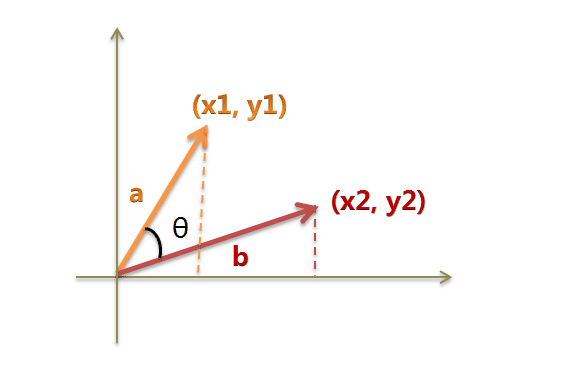$cos\Theta =\frac{{x}_{1}{x}_{2}+{y}_{1}{y}_{2}}{\sqrt{{x}_{1}^{2}+{y}_{1}^{2}}\ast \sqrt{{x}_{2}^{2}+{y}_{2}^{2}}}$$\large cos\varTheta = \frac{x_1x_2 + y_1y_2}{\sqrt{x_1^2 + y_1^2} * \sqrt{x_2^2 + y_2^2}}$

$cos\Theta =\frac{{\Sigma }_{i=1}^{n}\left({A}_{i}\ast {B}_{i}\right)}{\sqrt{{\Sigma }_{i=1}^{n}\left({A}_{i}{\right)}^{2}}\ast \sqrt{{\Sigma }_{i=1}^{n}\left({B}_{i}{\right)}^{2}}}=\frac{A\cdot B}{|A|\ast |B|}$$\large cos\varTheta = \frac{\varSigma_{i = 1}^n(A_i * B_i)}{\sqrt{\varSigma_{i = 1}^n(A_i)^2} * \sqrt{\varSigma_{i = 1}^n(B_i)^2}} = \frac{A \cdot B}{|A| * |B|}$

$cos\Theta =\frac{1\ast 1+2\ast 2+2\ast 2+1\ast 1+1\ast 1+1\ast 2+0\ast 1}{\sqrt{{1}^{2}+{2}^{2}+{2}^{2}+{1}^{2}+{1}^{2}+{1}^{2}+{0}^{2}}\ast \sqrt{{1}^{2}+{2}^{2}+{2}^{2}+{1}^{2}+{1}^{2}+{2}^{2}+{1}^{2}}}=\frac{13}{\sqrt{12}\ast \sqrt{16}}=0.938$$\large cos\varTheta = \frac{1 * 1 + 2 * 2 + 2* 2 + 1 * 1 + 1 * 1 + 1 * 2 + 0 * 1}{\sqrt{1^2 + 2^2 + 2^2 + 1^2 + 1^2 + 1^2 + 0^2} * \sqrt{1^2 + 2^2 + 2^2 + 1^2 + 1^2+ 2^2 + 1^2}} = \frac {13} {\sqrt{12} * \sqrt{16}} = 0.938$

def cosineSimilarity(vec1: DoubleMatrix, vec2: DoubleMatrix): Double = {
vec1.dot(vec2) / (vec1.norm2() * vec2.norm2())
}
import org.jblas.DoubleMatrix
val aMatrix = new DoubleMatrix(Array(1.0, 2.0, 3.0))

val sims = model.productFeatures.map{ case (id, factor) =>
val factorVector = new DoubleMatrix(factor)
val sim = cosineSimilarity(factorVector, itemVector)
(id, sim)
}

val sortedSims2 = sims.top(K + 1)(Ordering.by[(Int, Double), Double] { case (id, similarity) => similarity })
sortedSims2.slice(1, 11).map{ case (id, sim) => (titles(id), sim) }.mkString("\n")
/*
(Hideaway (1995),0.6932331537649621)
(Body Snatchers (1993),0.6898690594544726)
(Evil Dead II (1987),0.6897964975027041)
(Alien: Resurrection (1997),0.6891221044611473)
(Stephen King's The Langoliers (1995),0.6864214133620066)
(Liar Liar (1997),0.6812075443259535)
(Tales from the Crypt Presents: Bordello of Blood (1996),0.6754663844488256)
(Army of Darkness (1993),0.6702643811753909)
(Mystery Science Theater 3000: The Movie (1996),0.6594872765176396)
(Scream (1996),0.6538249646863378)
*/

# 4. ALS矩阵分解算法并行化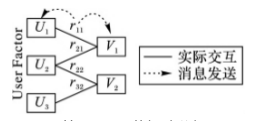（1）窄依赖（narrow dependencies）：子RDD的每个分区依赖于常数个父分区（即与数据规模无关）；
（2）宽依赖（wide dependencies）：子RDD的每个分区依赖于所有父RDD分区。例如，map产生窄依赖，而join则是宽依赖（除非父RDD被哈希分区）${}^{\left[4\right]}$$^{}$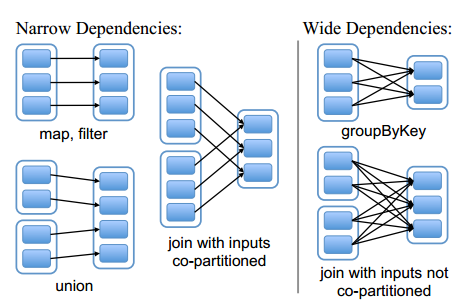# 5. ALS模型实现

val als = new ALS()
.setRank(12)
.setMaxIter(50)
.setRegParam(0.01)
.setUserCol("userId")
.setItemCol("movieId")
.setRatingCol("rating")
val model = als.fit(training)

val predictions = model.transform(test)

## 5.1 ALS.fit

 override def fit(dataset: Dataset[_]): ALSModel = {
transformSchema(dataset.schema)        //(1)
import dataset.sparkSession.implicits._

val r = if ($(ratingCol) != "") col($(ratingCol)).cast(FloatType) else lit(1.0f) //（2）
val ratings = dataset
.select(checkedCast(col($(userCol)).cast(DoubleType)), checkedCast(col($(itemCol)).cast(DoubleType)), r)
.rdd
.map { row =>
Rating(row.getInt(0), row.getInt(1), row.getFloat(2))
}                                      //（3）
val instrLog = Instrumentation.create(this, ratings) //（4）
instrLog.logParams(rank, numUserBlocks, numItemBlocks, implicitPrefs, alpha,
userCol, itemCol, ratingCol, predictionCol, maxIter,
regParam, nonnegative, checkpointInterval, seed)   //（5）
val (userFactors, itemFactors) = ALS.train(ratings, rank = $(rank), numUserBlocks =$(numUserBlocks), numItemBlocks = $(numItemBlocks), maxIter =$(maxIter), regParam = $(regParam), implicitPrefs =$(implicitPrefs),
alpha = $(alpha), nonnegative =$(nonnegative),
intermediateRDDStorageLevel = StorageLevel.fromString($(intermediateStorageLevel)), finalRDDStorageLevel = StorageLevel.fromString($(finalStorageLevel)),
checkpointInterval = $(checkpointInterval), seed =$(seed))  //（6）
val userDF = userFactors.toDF("id", "features")  //（7）
val itemDF = itemFactors.toDF("id", "features")  //（8）
val model = new ALSModel(uid, $(rank), userDF, itemDF).setParent(this) //（9） instrLog.logSuccess(model) //（10） copyValues(model) //（11） } (1)： transformSchema函数最终会调用validateAndTransformSchema将dataset的中的validateAndTransformSchema，去检查用户ID、物品ID、评分是否都为数值，并为dataset新增predictionCol的列，其类型为Float。 (2)(3)：将dataset将用户ID和商品ID转为Int类型，将评分转为Double类型，并生成Rating RDD。 (4)(5)：生成训练时打印log的仪器并设置参数。 (6)(7)(8)：训练模型得到用户特征矩阵userFactors和物品特征矩阵itemFactors，并对列重命名得到userDFitemDF (9)(10)(11)：传入rank, userDF, itemDF创建ALS模型。 ## 5.2 ALS.train 这一节我们来深入研究下，上节代码（6）中是如何计算出用户特征矩阵userFactors和物品特征矩阵itemFactors的。接下来，我们来逐行分析。 ### 5.2.1 参数 我们先来看下ALS.train的参数： ALS.train  def train[ID: ClassTag]( ratings: RDD[Rating[ID]], rank: Int = 10, numUserBlocks: Int = 10, numItemBlocks: Int = 10, maxIter: Int = 10, regParam: Double = 1.0, implicitPrefs: Boolean = false, alpha: Double = 1.0, nonnegative: Boolean = false, intermediateRDDStorageLevel: StorageLevel = StorageLevel.MEMORY_AND_DISK, finalRDDStorageLevel: StorageLevel = StorageLevel.MEMORY_AND_DISK, checkpointInterval: Int = 10, seed: Long = 0L)( implicit ord: Ordering[ID]): (RDD[(ID, Array[Float])], RDD[(ID, Array[Float])]) = { ...... } 以上定义中， • ratings指用户提供的训练数据，它包括用户id集、商品id集以及相应的打分集。  case class Rating[@specialized(Int, Long) ID](user: ID, item: ID, rating: Float) • rank表示隐含因素的数量，也即特征的数量。 • numUserBlocks和numItemBlocks分别指用户和商品的块数量，即分区数量。 • regParam表示最小二乘法中lambda值的大小。 • implicitPrefs表示我们的训练数据是否是隐式反馈数据。 • Nonnegative表示求解的最小二乘的值是否是非负,根据Nonnegative的值的不同，spark使用了不同的求解方法。 ### 5.2.2 初始化ALSPartitioner和LocalIndexEncoder ALS.train  require(intermediateRDDStorageLevel != StorageLevel.NONE, "ALS is not designed to run without persisting intermediate RDDs.") val sc = ratings.sparkContext val userPart = new ALSPartitioner(numUserBlocks) val itemPart = new ALSPartitioner(numItemBlocks) val userLocalIndexEncoder = new LocalIndexEncoder(userPart.numPartitions) val itemLocalIndexEncoder = new LocalIndexEncoder(itemPart.numPartitions) • ALSPartitioner实现了基于hash的分区，它根据用户或者商品id的hash值来进行分区。ALSPartitioner即HashPartitioner：  private[recommendation] type ALSPartitioner = org.apache.spark.HashPartitioner class HashPartitioner(partitions: Int) extends Partitioner { require(partitions >= 0, s"Number of partitions ($partitions) cannot be negative.")

def numPartitions: Int = partitions

def getPartition(key: Any): Int = key match {
case null => 0
case _ => Utils.nonNegativeMod(key.hashCode, numPartitions)
}

override def equals(other: Any): Boolean = other match {
case h: HashPartitioner =>
h.numPartitions == numPartitions
case _ =>
false
}

override def hashCode: Int = numPartitions
}
• LocalIndexEncoder（blockid，localindex）即（分区id，分区内索引）进行编码，并将其转换为一个整数，这个整数在高位存分区ID，在低位存对应分区的索引，在空间上尽量做到了不浪费。同时也可以根据这个转换的整数分别获得blockid和localindex。这两个对象在后续的代码中会用到。
  private[recommendation] class LocalIndexEncoder(numBlocks: Int) extends Serializable {

require(numBlocks > 0, s"numBlocks must be positive but found $numBlocks.") private[this] final val numLocalIndexBits = math.min(java.lang.Integer.numberOfLeadingZeros(numBlocks - 1), 31) private[this] final val localIndexMask = (1 << numLocalIndexBits) - 1 /** 将一个(blockId, localIndex) 在一个 integer 中编码 */ def encode(blockId: Int, localIndex: Int): Int = { require(blockId < numBlocks) require((localIndex & ~localIndexMask) == 0) (blockId << numLocalIndexBits) | localIndex } /** 从编码的index得到 block id */ @inline def blockId(encoded: Int): Int = { encoded >>> numLocalIndexBits } /** 从编码的index得到 local index */ @inline def localIndex(encoded: Int): Int = { encoded & localIndexMask } } ### 5.2.3 根据nonnegative参数选择解决矩阵分解的方法 ALS.train  val solver = if (nonnegative) new NNLSSolver else new CholeskySolver 如果需要解的值为非负,即nonnegativetrue，那么用非负最小二乘（NNLS）来解，如果没有这个限制，用乔里斯基（Cholesky）分解来解。优化器的工作原理我们会在后续讲解。 ### 5.2.4 将ratings数据转换为分区的格式 ALS.train  val blockRatings = partitionRatings(ratings, userPart, itemPart) .persist(intermediateRDDStorageLevel) 将ratings数据转换为分区的形式，即（（用户分区id，商品分区id），分区数据集blocks））的形式，并缓存到内存中。其中分区id的计算是通过ALSPartitionergetPartitions方法获得的，分区数据集由RatingBlock组成， 它表示（用户分区id，商品分区id ）对所对应的用户id集，商品id集，以及打分集，即（用户id集，商品id集，打分集）。 我们来看下partitionRatings  private def partitionRatings[ID: ClassTag]( ratings: RDD[Rating[ID]], srcPart: Partitioner, dstPart: Partitioner): RDD[((Int, Int), RatingBlock[ID])] = { // 计算总共的分区数，默认的 10 × 10 val numPartitions = srcPart.numPartitions * dstPart.numPartitions // 对ratings的每个分区进行操作 ratings.mapPartitions { iter => // 用numPartitions个RatingBlockBuilder[ID]一维数组来模拟RatingBlockBuilder[ID]矩阵 val builders = Array.fill(numPartitions)(new RatingBlockBuilder[ID]) // 对同一分区中每个ratings值操作 iter.flatMap { r => // 得到用户分区id val srcBlockId = srcPart.getPartition(r.user) // 得到商品分区id val dstBlockId = dstPart.getPartition(r.item) // 计算出对应的RatingBlockBuilder[ID]数组中的id val idx = srcBlockId + srcPart.numPartitions * dstBlockId // 得到该 RatingBlockBuilder val builder = builders(idx) // 将 该 ratings值 加入 该builder builder.add(r) // 若该builder>=2048 if (builder.size >= 2048) { // 2048 * (3 * 4) = 24k // builders(idx) = new RatingBlockBuilder // 得到结果 Iterator.single(((srcBlockId, dstBlockId), builder.build())) } else { Iterator.empty } } ++ { //上步结束后，若builders有还存在着ratings值builder builders.view.zipWithIndex.filter(_._1.size > 0).map { case (block, idx) => // 根据 RatingBlockBuilder[ID]数组中的id 反推出 用户分区id和商品分区id val srcBlockId = idx % srcPart.numPartitions val dstBlockId = idx / srcPart.numPartitions // 得到结果 ((srcBlockId, dstBlockId), block.build()) } } // 根据 （用户分区id，商品分区id） 为key 进行聚合 // 然后对 每个值Value 中的 blocks 操作 }.groupByKey().mapValues { blocks => val builder = new RatingBlockBuilder[ID] // 将每个block 合并 到 builder blocks.foreach(builder.merge) // 得到合并后的结果 builder.build() }.setName("ratingBlocks") } ### 5.2.5 获取inblocks和outblocks数据 我们知道，通信复杂度是分布式实现一个算法时要重点考虑的问题，不同的实现可能会对性能产生很大的影响。我们假设最坏的情况：即求解商品需要的所有用户特征都需要从其它节点获得。 如下图所示：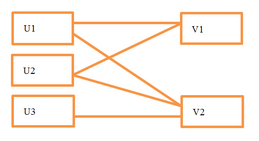求解v1需要获得u1,u2，求解v2需要获得u1,u2,u3等，在这种假设下，每步迭代所需的交换数据量是O(m*rank)，其中m表示所有观察到的打分集大小，rank表示特征数量。 我们知道，如果计算v1v2是在同一个分区上进行的，那么我们只需要把u1u2一次发给这个分区就好了，而不需要将u1u2分别发给v1,v2，这样就省掉了不必要的数据传输。 下图描述了如何在分区的情况下通过U来求解V，注意节点之间的数据交换量减少了：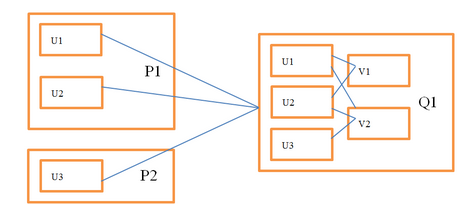使用这种分区结构，我们需要在原始打分数据的基础上额外保存一些信息。 Q1中，我们需要知道和v1相关联的用户向量及其对应的打分，从而构建最小二乘问题并求解。这部分数据不仅包含原始打分数据，还包含从每个用户分区收到的向量排序信息，在代码里称作InBlock P1中，我们要知道把u1,u2发给Q1。我们可以查看和u1相关联的所有产品来确定需要把u1发给谁，但每次迭代都扫一遍数据很不划算，所以在spark的实现中只计算一次这个信息，然后把结果通过RDD缓存起来重复使用。这部分数据我们在代码里称作OutBlock 所以从U求解V，我们需要通过用户的OutBlock信息把用户向量发给商品分区，然后通过商品的InBlock信息构建最小二乘问题并求解。从V求解U，我们需要商品的OutBlock信息和用户的InBlock信息。所有的InBlockOutBlock信息在迭代过程中都通过RDD缓存。打分数据在用户的InBlock和商品的InBlock各存了一份，但分区方式不同。这么做可以避免在迭代过程中原始数据的交换。 下面介绍获取InBlockOutBlock的方法。下面的代码用来分别获取用户和商品的InBlockOutBlock ALS.train  val (userInBlocks, userOutBlocks) = makeBlocks("user", blockRatings, userPart, itemPart, intermediateRDDStorageLevel) userOutBlocks.count() // 交换userBlockId和itemBlockId以及其对应的数据 val swappedBlockRatings = blockRatings.map { case ((userBlockId, itemBlockId), RatingBlock(userIds, itemIds, localRatings)) => ((itemBlockId, userBlockId), RatingBlock(itemIds, userIds, localRatings)) } val (itemInBlocks, itemOutBlocks) = makeBlocks("item", swappedBlockRatings, itemPart, userPart, intermediateRDDStorageLevel) itemOutBlocks.count() 我们会以求商品的InBlock以及用户的OutBlock为例来分析makeBlocks方法。 #### 5.2.5.1 InBlock 下面以求商品的InBlock为例子，则src将代表商品，dst代表着用户。看下makeBlocks方法中的InBlock部分：  val inBlocks = ratingBlocks.map { // 这部分代码工作主要是： // 将用户id映射为该分区中的用户id。 case ((srcBlockId, dstBlockId), RatingBlock(srcIds, dstIds, ratings)) => val start = System.nanoTime() // 用 OpenHashSet 对用户id去重 val dstIdSet = new OpenHashSet[ID](1 << 20) dstIds.foreach(dstIdSet.add) val sortedDstIds = new Array[ID](dstIdSet.size) var i = 0 var pos = dstIdSet.nextPos(0) while (pos != -1) { sortedDstIds(i) = dstIdSet.getValue(pos) pos = dstIdSet.nextPos(pos + 1) i += 1 } assert(i == dstIdSet.size) // 将用户id 排序 Sorting.quickSort(sortedDstIds) val dstIdToLocalIndex = new OpenHashMap[ID, Int](sortedDstIds.length) i = 0 // 建立用户id与分区中的用户id（从0开始：0,1,2...）的映射关系 while (i < sortedDstIds.length) { dstIdToLocalIndex.update(sortedDstIds(i), i) i += 1 } logDebug( "Converting to local indices took " + (System.nanoTime() - start) / 1e9 + " seconds.") //将用户id 映射为 该分区中的用户id val dstLocalIndices = dstIds.map(dstIdToLocalIndex.apply) // 返回结果为：（商品分区id，（用户分区id，商品id集合，分区中对应的用户id集合，打分集合） (srcBlockId, (dstBlockId, srcIds, dstLocalIndices, ratings)) // srcPart的分区进行聚合 }.groupByKey(new ALSPartitioner(srcPart.numPartitions)) .mapValues { iter => ...... 可见spark代码实现中，并没有存储用户的真实id，而是存储的使用LocalIndexEncoder生成的编码，这样节省了空间，格式为UncompressedInBlock:（商品id集合，分区中对应的用户id集合，打分集合）， 如： ([v1, v2, v1, v2, v2], [ui1, ui1, ui2, ui2, ui3], [r11, r12, r21, r22, r32]) 这种结构仍旧有压缩的空间，spark调用compress方法将商品id进行排序（排序有两个好处，除了压缩以外，后文构建最小二乘也会因此受益）， 并且转换为（不重复的有序的商品id集合，商品位置偏移集合，分区中对应的用户id集合，打分集合）的形式，以获得更优的存储效率（代码中就是将矩阵的coo格式转换为csc格式，你可以更进一步了解矩阵存储，以获得更多信息）。 以这样的格式修改： ([v1, v2, v1, v2, v2], [ui1, ui1, ui2, ui2, ui3], [r11, r12, r21, r22, r32]) 排序后得到： ([v1, v1 v2, v2, v2], [ui1, ui1, ui2, ui2, ui3], [r11, r12, r21, r22, r32]) 最终得到的结果是： ([v1, v2], [0, 2, 5], [ui1, ui2, ui1, ui2, ui3], [r11, r21, r12, r22, r32]) 其中[0, 2]v1对应的打分的位置区间是[0, 2），v2对应的打分的位置区间是[2, 5） 我们继续看mapValues中的代码 ：  .mapValues { iter => val builder = new UncompressedInBlockBuilder[ID](new LocalIndexEncoder(dstPart.numPartitions)) iter.foreach { case (dstBlockId, srcIds, dstLocalIndices, ratings) => builder.add(dstBlockId, srcIds, dstLocalIndices, ratings) } builder.build().compress() }.setName(prefix + "InBlocks") .persist(storageLevel) 下面我们就来看下compress方法是如何创建InBlock的：  def compress(): InBlock[ID] = { val sz = length assert(sz > 0, "Empty in-link block should not exist.") // 商品id进行排序 sort() val uniqueSrcIdsBuilder = mutable.ArrayBuilder.make[ID] val dstCountsBuilder = mutable.ArrayBuilder.make[Int] var preSrcId = srcIds(0) uniqueSrcIdsBuilder += preSrcId var curCount = 1 var i = 1 // 笔者认为这里的变量j是无用的 var j = 0 while (i < sz) { val srcId = srcIds(i) if (srcId != preSrcId) { uniqueSrcIdsBuilder += srcId dstCountsBuilder += curCount preSrcId = srcId j += 1 curCount = 0 } curCount += 1 i += 1 } dstCountsBuilder += curCount val uniqueSrcIds = uniqueSrcIdsBuilder.result() val numUniqueSrdIds = uniqueSrcIds.length val dstCounts = dstCountsBuilder.result() val dstPtrs = new Array[Int](numUniqueSrdIds + 1) var sum = 0 i = 0 while (i < numUniqueSrdIds) { // 计算偏移 sum += dstCounts(i) i += 1 dstPtrs(i) = sum } // 返回值InBlock中包含 （不重复的有序的商品id集合，商品位置偏移集合，分区中对应的用户id集合，打分集合） InBlock(uniqueSrcIds, dstPtrs, dstEncodedIndices, ratings) } 笔者认为上述的变量j是无用的  var j = 0 该问题已经提交到Spark Github 并merged：https://github.com/apache/spark/commit/cca8680047bb2ec312ffc296a561abd5cbc8323c #### 5.2.5.2 OutBlock 下面以求用户的OutBlock为例子，则src将代表用户，dst代表着商品。看下makeBlocks方法中的OutBlock部分： // 这里的inBlocks的格式为 // （用户分区id，（不重复的有序的用户id集合，用户位置偏移集合，分区中对应的商品id集合，打分集合）） val outBlocks = inBlocks.mapValues { case InBlock(srcIds, dstPtrs, dstEncodedIndices, _) => val encoder = new LocalIndexEncoder(dstPart.numPartitions) // activeIds是一个二维数组 // 第一维是 商品的分区id // 第二位是 不重复的有序的用户id集合的位置的集合 val activeIds = Array.fill(dstPart.numPartitions)(mutable.ArrayBuilder.make[Int]) var i = 0 // 用来记录 该用户的id是否已经加入activeIds了各个第一维度（商品的分区）中 val seen = new Array[Boolean](dstPart.numPartitions) // 遍历不重复的有序的用户id集合 while (i < srcIds.length) { // 遍历用户id为i的用户所对应的所有商品id var j = dstPtrs(i) // 置全为否 ju.Arrays.fill(seen, false) while (j < dstPtrs(i + 1)) { // 根据商品id得到商品分区id val dstBlockId = encoder.blockId(dstEncodedIndices(j)) if (!seen(dstBlockId)) { // 该不重复的有序的用户id集合的位置 加入activeIds对应的商品分区下 activeIds(dstBlockId) += i seen(dstBlockId) = true } j += 1 } i += 1 } activeIds.map { x => x.result() } }.setName(prefix + "OutBlocks") .persist(storageLevel) ### 5.2.6 利用inblock和outblock信息构建最小二乘 交换最小二乘算法是分别固定用户特征矩阵和商品特征矩阵来交替计算下一次迭代的商品特征矩阵和用户特征矩阵 初始化后的userFactors的格式是（用户分区id，用户特征矩阵factors），其中factors是一个二维数组，第一维的长度是用户数，第二维的长度是rank数。初始化的值是异或随机数的F范式。itemFactors的初始化与此类似。 通过用户的OutBlock把用户信息发给商品分区，然后结合商品的InBlock信息构建最小二乘问题，我们就可以借此解得商品的极小解。反之，通过商品OutBlock把商品信息发送给用户分区，然后结合用户的InBlock信息构建最小二乘问题，我们就可以解得用户解： ALS.train  val seedGen = new XORShiftRandom(seed) var userFactors = initialize(userInBlocks, rank, seedGen.nextLong()) var itemFactors = initialize(itemInBlocks, rank, seedGen.nextLong()) var previousCheckpointFile: Option[String] = None val shouldCheckpoint: Int => Boolean = (iter) => sc.checkpointDir.isDefined && checkpointInterval != -1 && (iter % checkpointInterval == 0) val deletePreviousCheckpointFile: () => Unit = () => previousCheckpointFile.foreach { file => try { val checkpointFile = new Path(file) checkpointFile.getFileSystem(sc.hadoopConfiguration).delete(checkpointFile, true) } catch { case e: IOException => logWarning(s"Cannot delete checkpoint file$file:", e)
}
}
// 隐式偏好
if (implicitPrefs) {
for (iter <- 1 to maxIter) {
userFactors.setName(s"userFactors-$iter").persist(intermediateRDDStorageLevel) val previousItemFactors = itemFactors itemFactors = computeFactors(userFactors, userOutBlocks, itemInBlocks, rank, regParam, userLocalIndexEncoder, implicitPrefs, alpha, solver) previousItemFactors.unpersist() itemFactors.setName(s"itemFactors-$iter").persist(intermediateRDDStorageLevel)
val deps = itemFactors.dependencies
if (shouldCheckpoint(iter)) {
itemFactors.checkpoint()
}
val previousUserFactors = userFactors
userFactors = computeFactors(itemFactors, itemOutBlocks, userInBlocks, rank, regParam,
itemLocalIndexEncoder, implicitPrefs, alpha, solver)
if (shouldCheckpoint(iter)) {
ALS.cleanShuffleDependencies(sc, deps)
deletePreviousCheckpointFile()
previousCheckpointFile = itemFactors.getCheckpointFile
}
previousUserFactors.unpersist()
}
// 显示偏好
} else {
for (iter <- 0 until maxIter) {
itemFactors = computeFactors(userFactors, userOutBlocks, itemInBlocks, rank, regParam,
userLocalIndexEncoder, solver = solver)
if (shouldCheckpoint(iter)) {
val deps = itemFactors.dependencies
itemFactors.checkpoint()
itemFactors.count()
ALS.cleanShuffleDependencies(sc, deps)
deletePreviousCheckpointFile()
previousCheckpointFile = itemFactors.getCheckpointFile
}
userFactors = computeFactors(itemFactors, itemOutBlocks, userInBlocks, rank, regParam,
itemLocalIndexEncoder, solver = solver)
}
}
// 生成用户特征矩阵
val userIdAndFactors = userInBlocks
.mapValues(_.srcIds)
.join(userFactors)
.mapPartitions({ items =>
items.flatMap { case (_, (ids, factors)) =>
ids.view.zip(factors)
}
}, preservesPartitioning = true)
.setName("userFactors")
.persist(finalRDDStorageLevel)
// 生成商品特征矩阵
val itemIdAndFactors = itemInBlocks
.mapValues(_.srcIds)
.join(itemFactors)
.mapPartitions({ items =>
items.flatMap { case (_, (ids, factors)) =>
ids.view.zip(factors)
}
}, preservesPartitioning = true)
.setName("itemFactors")
.persist(finalRDDStorageLevel)
if (finalRDDStorageLevel != StorageLevel.NONE) {
userIdAndFactors.count()
itemFactors.unpersist()
itemIdAndFactors.count()
userInBlocks.unpersist()
userOutBlocks.unpersist()
itemInBlocks.unpersist()
itemOutBlocks.unpersist()
blockRatings.unpersist()
}
(userIdAndFactors, itemIdAndFactors)
}

#### 5.2.6.1 computeFactors

  private def computeFactors[ID](
srcFactorBlocks: RDD[(Int, FactorBlock)],
srcOutBlocks: RDD[(Int, OutBlock)],
dstInBlocks: RDD[(Int, InBlock[ID])],
rank: Int,
regParam: Double,
srcEncoder: LocalIndexEncoder,
implicitPrefs: Boolean = false,
alpha: Double = 1.0,
solver: LeastSquaresNESolver): RDD[(Int, FactorBlock)] = {
val numSrcBlocks = srcFactorBlocks.partitions.length
// 若隐式偏好
// 则计算 YtY
val YtY = if (implicitPrefs) Some(computeYtY(srcFactorBlocks, rank)) else None
val srcOut =
// 用用户outblock与userFactor进行join操作
srcOutBlocks.join(srcFactorBlocks).flatMap {
case (srcBlockId, (srcOutBlock, srcFactors)) =>
srcOutBlock.view.zipWithIndex.map { case (activeIndices, dstBlockId) =>
// 每一个商品分区包含一组所需的用户分区及其对应的用户factor信息
// 格式即（商品分区id集合，（用户分区id集合，用户分区对应的factor集合））
(dstBlockId, (srcBlockId, activeIndices.map(idx => srcFactors(idx))))
}
}

// 以商品分区id为key进行分组
val merged = srcOut.groupByKey(new ALSPartitioner(dstInBlocks.partitions.length))
// 用商品inblock信息与merged进行join操作
dstInBlocks.join(merged).mapValues {
......

......
// 遍历各个商品分区
// 数据格式为 （（该商品分区的不重复的有序的商品id集合，该商品分区的商品位置偏移集合，该商品分区中对应的用户id集合，打分集合），（用户分区id集合->用户分区对应的factor集合））
case (InBlock(dstIds, srcPtrs, srcEncodedIndices, ratings), srcFactors) =>
// 从 srcFactors: Iterable[(Int,Array[Array[Float]])] 中取出值到数组sortedSrcFactors
// 即得得到用户特征数组
val sortedSrcFactors = new Array[FactorBlock](numSrcBlocks)
srcFactors.foreach { case (srcBlockId, factors) =>
sortedSrcFactors(srcBlockId) = factors
}
// 保存要求解的商品特征
val dstFactors = new Array[Array[Float]](dstIds.length)
var j = 0
// 保存rank维度的正规方程组
val ls = new NormalEquation(rank)
// 遍历商品
while (j < dstIds.length) {
ls.reset()
if (implicitPrefs) {
ls.merge(YtY.get)
}
var i = srcPtrs(j)
var numExplicits = 0
// 商品dstIds(j)对应的遍历用户
while (i < srcPtrs(j + 1)) {
// 解码得到用户分区号 和 该分区内的地址
val encoded = srcEncodedIndices(i)
val blockId = srcEncoder.blockId(encoded)
val localIndex = srcEncoder.localIndex(encoded)
// 得到 该用户特征向量
val srcFactor = sortedSrcFactors(blockId)(localIndex)
val rating = ratings(i)
if (implicitPrefs) {
val c1 = alpha * math.abs(rating)
if (rating > 0) {
numExplicits += 1
ls.add(srcFactor, (c1 + 1.0) / c1, c1)
}
} else {
ls.add(srcFactor, rating)
numExplicits += 1
}
i += 1
}
// 优化商品dstIds(j)对应的特征向量
dstFactors(j) = solver.solve(ls, numExplicits * regParam)
j += 1
}
dstFactors
}
}


### 5.2.7 优化器

  private[recommendation] trait LeastSquaresNESolver extends Serializable {
def solve(ne: NormalEquation, lambda: Double): Array[Float]
}

CholeskySolver和NNLSSolver实现了LeastSquaresNESolver。

#### 5.2.7.1 CholeskySolver

  private[recommendation] class CholeskySolver extends LeastSquaresNESolver {

/**
* 这里所使用的代价函数为：
*   $min norm(A x - b)^2^ + lambda * norm(x)^2^$
*/
override def solve(ne: NormalEquation, lambda: Double): Array[Float] = {
val k = ne.k
// 在上正定矩阵A的对角线上加上lambda
var i = 0
var j = 2
while (i < ne.triK) {
ne.ata(i) += lambda
i += j
j += 1
}
//直接调用netlib-java封装的方法实现
CholeskyDecomposition.solve(ne.ata, ne.atb)
val x = new Array[Float](k)
i = 0
while (i < k) {
x(i) = ne.atb(i).toFloat
i += 1
}
ne.reset()
x
}
}

#### 5.2.7.2 NNLSSolver

  private[recommendation] class NNLSSolver extends LeastSquaresNESolver {
private var rank: Int = -1
private var workspace: NNLS.Workspace = _
private var ata: Array[Double] = _
private var initialized: Boolean = false

private def initialize(rank: Int): Unit = {
if (!initialized) {
this.rank = rank
workspace = NNLS.createWorkspace(rank)
ata = new Array[Double](rank * rank)
initialized = true
} else {
require(this.rank == rank)
}
}

/**
* Solves a nonnegative least squares problem with L2 regularization:
*
*   min_x_  norm(A x - b)^2^ + lambda * n * norm(x)^2^
*   subject to x >= 0
*/
override def solve(ne: NormalEquation, lambda: Double): Array[Float] = {
val rank = ne.k
initialize(rank)
fillAtA(ne.ata, lambda)
val x = NNLS.solve(ata, ne.atb, workspace)
ne.reset()
x.map(x => x.toFloat)
}

/**
* Given a triangular matrix in the order of fillXtX above, compute the full symmetric square
* matrix that it represents, storing it into destMatrix.
*/
private def fillAtA(triAtA: Array[Double], lambda: Double) {
var i = 0
var pos = 0
var a = 0.0
while (i < rank) {
var j = 0
while (j <= i) {
a = triAtA(pos)
ata(i * rank + j) = a
ata(j * rank + i) = a
pos += 1
j += 1
}
ata(i * rank + i) += lambda
i += 1
}
}
}


# 6. 参考文献

【1】Y Koren, R Bell, C Volinsky. Matrix factorization techniques for recommender systems. IEEE, Computer Journal,2009: 42(8), 30-37
【2】ZHENG F. Matrix factorization recommendation algorithm based on Spark [D]. Chengdu: School of Information Science and Technology, Southwest Jiaotong University, 2015.
【3】Yang Z.The Research of Recommendation System Based on Spark Platform [D].Anhui:University of Science and Technology of China,2015.
【4】M Zaharia，M Chowdhury，T Das，A Dave，J Ma . Resilient Distributed Datasets: A Fault-Tolerant Abstraction for In-Memory Cluster Computing. Usenix Conference on Networked Systems Design, 2012, 70(2):141-146
【5】《交换最小二乘》